# KR100561869B1 - Lossless audio decoding/encoding method and apparatus - Google Patents

## Info

Publication number
KR100561869B1
KR100561869B1 KR1020040050479A KR20040050479A KR100561869B1 KR 100561869 B1 KR100561869 B1 KR 100561869B1 KR 1020040050479 A KR1020040050479 A KR 1020040050479A KR 20040050479 A KR20040050479 A KR 20040050479A KR 100561869 B1 KR100561869 B1 KR 100561869B1
Authority
KR
South Korea
Prior art keywords
frequency
context
binary sample
audio
importance
Prior art date
Application number
KR1020040050479A
Other languages
Korean (ko)
Other versions
KR20050090941A (en
Inventor
김상욱
김중회
미아오레이
오은미
이시화
Original Assignee
삼성전자주식회사
Priority date (The priority date is an assumption and is not a legal conclusion. Google has not performed a legal analysis and makes no representation as to the accuracy of the date listed.)
Filing date
Publication date
Priority to US55135904P priority Critical
Priority to US60/551,359 priority
Application filed by 삼성전자주식회사 filed Critical 삼성전자주식회사
Priority to KR1020040050479A priority patent/KR100561869B1/en
Publication of KR20050090941A publication Critical patent/KR20050090941A/en
Application granted granted Critical
Publication of KR100561869B1 publication Critical patent/KR100561869B1/en

• 230000003595 spectral Effects 0.000 claims abstract description 38
• 238000007906 compression Methods 0.000 claims abstract description 35
• 238000004364 calculation methods Methods 0.000 claims description 24
• 238000006243 chemical reaction Methods 0.000 claims description 14
• 230000000875 corresponding Effects 0.000 claims description 7
• 230000002194 synthesizing Effects 0.000 claims description 6
• 230000001131 transforming Effects 0.000 claims 1
• 230000000694 effects Effects 0.000 description 8
• 238000000034 methods Methods 0.000 description 3
• 238000007619 statistical methods Methods 0.000 description 2

## Images

•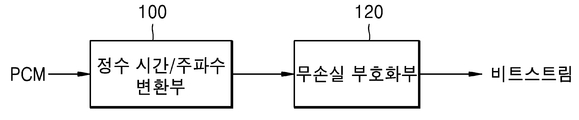•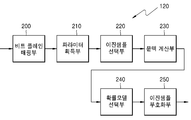•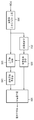•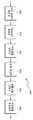•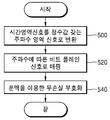•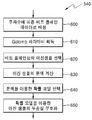•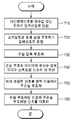•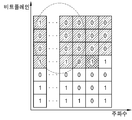•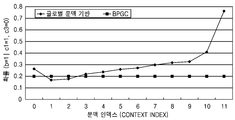•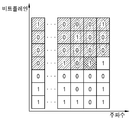•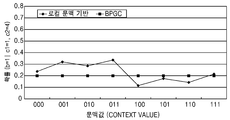•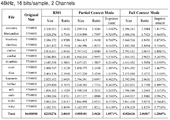•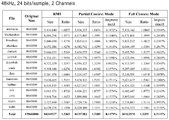•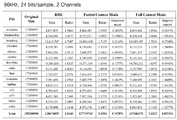•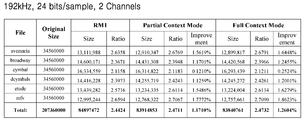••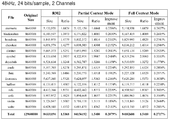•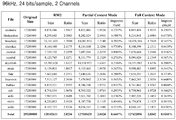••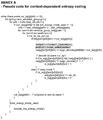•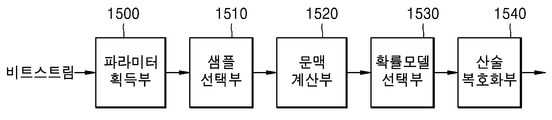•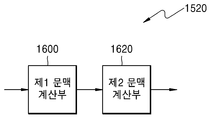•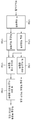•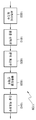•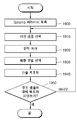•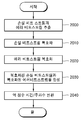## Classifications

• GPHYSICS
• G10MUSICAL INSTRUMENTS; ACOUSTICS
• G10LSPEECH ANALYSIS OR SYNTHESIS; SPEECH RECOGNITION; SPEECH OR VOICE PROCESSING; SPEECH OR AUDIO CODING OR DECODING
• G10L19/00Speech or audio signals analysis-synthesis techniques for redundancy reduction, e.g. in vocoders; Coding or decoding of speech or audio signals, using source filter models or psychoacoustic analysis
• G10L19/0017Lossless audio signal coding; Perfect reconstruction of coded audio signal by transmission of coding error

## Abstract

The present invention relates to a lossless audio encoding / decoding method and apparatus, comprising: converting an audio signal in a time domain into an audio spectral signal in a frequency domain having an integer value; Mapping an audio signal in a frequency domain to a bitplane signal according to frequency; Obtaining a most significant bit and a Golomb parameter for each bit plane; Selecting a binary sample on the bitplane to be encoded in order from most significant bit to least significant bit, and then from low frequency component to high frequency component; Calculating the context of the selected binary sample using the importance of the already encoded bitplanes for each of the plurality of frequency lines present around the frequency line to which the selected binary sample belongs; Selecting a probabilistic model using the obtained Golomb parameter and the calculated contexts; And lossless encoding the binary sample using the selected probability model. According to the present invention, a compression ratio superior to BPGC is provided through a context-based encoding scheme having more optimal performance.

## Description

Lossless audio decoding / encoding method and apparatus

1 is a block diagram illustrating a configuration of an embodiment of a lossless audio encoding apparatus according to the present invention.

2 is a block diagram illustrating a configuration of a lossless encoding unit.

3 is a block diagram showing the configuration of another embodiment of a lossless audio encoding apparatus according to the present invention.

4 is a block diagram illustrating a configuration of the lossless encoding unit of FIG. 3.

FIG. 5 is a flowchart illustrating an operation of an embodiment of a lossless audio encoding apparatus according to the present invention shown in FIG. 1.

6 is a flowchart illustrating an operation of the lossless encoding unit illustrated in FIG. 1.

FIG. 7 is a flowchart illustrating an operation of another embodiment of the lossless audio encoding apparatus according to the present invention shown in FIG. 3.

8 is a diagram illustrating a global context in a context calculation unit.

9 is a graph illustrating a probability of 1 in the global context calculation in the context calculation unit.

10 is a diagram illustrating a local context in a context calculator.

11 is a graph illustrating a probability of 1 when calculating a local context in the context calculating unit.

12A to 12D are diagrams showing the size, compression ratio and improvement effect in RM1, local context and global context according to four experimental conditions.

13A to 13D are diagrams showing the size, compression ratio and improvement effect in RM2, local context and global context according to four experimental conditions.

Figure 14 shows one form of pseudo code for the present invention context-based coding.

15 is a block diagram illustrating a configuration of an embodiment of a lossless audio decoding apparatus according to the present invention.

FIG. 16 is a block diagram illustrating a configuration of the context calculator shown in FIG. 15.

17 is a block diagram illustrating a configuration of another embodiment of a lossless audio decoding apparatus according to the present invention.

18 is a block diagram showing the configuration of a lossless decoding unit.

19 is a flowchart illustrating an operation of an embodiment of a lossless audio decoding apparatus according to the present invention shown in FIG. 15.

20 is a flowchart illustrating an operation of another embodiment of the lossless audio decoding apparatus according to the present invention shown in FIG. 15.

The present invention relates to audio signal encoding / decoding, and more particularly, to a lossless audio encoding / decoding method and apparatus capable of providing a compression ratio superior to BPGC through a context-based encoding scheme.

Lossless audio coding methods include MLP (Meridian Lossless Audio Compression), Monkey's Audio, Free Lossless Audio Coding, and MLP is applied to DVD-A. As the network bandwidth of the Internet increases, a large amount of multimedia content is provided, and audio requires a lossless audio method. In the EU, digital audio broadcasting has already begun through DAB (Digital Audio Broadcasting), and broadcasting stations and content providers are using audio lossless coding. In the MPEG group, standardization of lossless audio compression is underway under the name of ISO / IEC 14496-3: 2001 / AMD 5, Audio Scalable to Lossless Coding (SLS). It provides Fine Grain Scalablity (FGS) and allows lossless audio compression.

Compression rate, the most important factor in lossless audio compression technology, can be improved by eliminating redundant information between data. The duplicated information may be removed through prediction between adjacent data or may be removed through a context between adjacent data.

Integer MDCT coefficients show a Laplacian distribution, in which the best known compression method, called the Golomb code, is used. Bitplane coding is required to provide FGS. The combination of Golomb code and bitplane coding is called Bit Plane Golomb Coding (BPGC), which provides an optimal compression rate and FGS. However, the assumption that the integer MDCT coefficients have a Laplacian distribution may not be appropriate for the actual data distribution. Since the BPGC is an algorithm designed on the assumption that the integer MDCT coefficients have a Laplacian distribution, the BPGC cannot provide an optimal compression ratio when the integer MDCT coefficients do not have a Laplacian distribution. Therefore, there is a need for a lossless audio coding and decoding method that can provide an optimal compression ratio regardless of the assumption that the integer MDCT coefficients have a Laplacian distribution.

An object of the present invention is to provide a lossless audio encoding method and apparatus capable of providing a more optimal compression ratio regardless of the assumption that integer MDCT coefficients have a Laplacian distribution.

According to an aspect of the present invention, there is provided a lossless audio encoding method comprising: converting an audio signal in a time domain into an audio spectral signal in a frequency domain having an integer value; Mapping the audio signal in the frequency domain to a bitplane signal according to frequency; Obtaining a most significant bit and a Golomb parameter for each bit plane; Selecting a binary sample on the bitplane to be encoded in order from most significant bit to least significant bit, then from low frequency component to high frequency component; Calculating a context of the selected binary sample using the importance of the already encoded bitplanes for each of a plurality of frequency lines that exist around the frequency line to which the selected binary sample belongs; Selecting a probabilistic model using the obtained Golomb parameter and the calculated contexts; And lossless encoding the binary sample using the selected probability model.

According to an aspect of the present invention, there is provided a lossless audio encoding method comprising: (a) converting an audio signal in a time domain into an audio spectral signal in a frequency domain having an integer value; (b) matching the audio spectral signal in the frequency domain with an input signal of a lossy encoder through scaling; (c) lossy compression coding the scaled frequency signal; (d) obtaining an error mapped signal corresponding to a difference between the lossy coded data and an audio spectral signal in the frequency domain having the integer value; (e) lossless encoding the error mapped signal using a context obtained based on the importance of the already encoded bitplanes for each of the plurality of frequency lines that exist around the frequency line to which the error mapped signal belongs; And (f) multiplexing the lossless coded signal and the lossy coded signal into a bitstream. Step (e) comprises: (e1) mapping the error mapped signal of step (d) to bitplane data according to frequency; (e2) obtaining the most significant bit and the Golomb parameter of the bitplane; (e3) selecting a binary sample on a bitplane to be encoded in order from most significant bit to least significant bit, and then from low frequency component to high frequency; (e4) calculating a context of the selected binary sample using the importance of the already encoded bitplanes for each of the plurality of frequency lines that exist around the frequency line to which the selected binary sample belongs; (e5) selecting a probabilistic model using the obtained Golomb parameter and the calculated contexts; And (e6) lossless encoding the binary sample using the selected probability model.

In the step (e4), the importance value of the already coded bitplanes samples on the same frequency lines in the plurality of frequency lines existing in front of the frequency line to which the selected binary sample belongs is obtained and binarized to determine the context value of the binary sample. It is preferable to calculate. Also, the step (e4) calculates the importance of the already coded bitplanes samples on the same frequency lines in the plurality of frequency lines located around the frequency line to which the selected binary sample belongs, and among the plurality of frequency lines. It is preferable to multiply a predetermined integer value by a ratio of how many frequency lines have importance, and then express the integer value using the integer.

According to an aspect of the present invention, there is provided a lossless audio encoding apparatus comprising: an integer time / frequency conversion unit converting an audio signal in a time domain into an audio spectral signal in a frequency domain having an integer value; A bitplane mapping unit for mapping the audio signal in the frequency domain into bitplane data according to frequency; A parameter obtaining unit for obtaining a most significant bit and a Golomb parameter of the bit plane; A binary sample selecting unit which selects a binary sample on a bitplane to be encoded in order from most significant bit to least significant bit, and then from lowest frequency component to highest frequency; A context calculator configured to calculate a context of the selected binary sample by using importance of bitplanes that are already encoded for each of a plurality of frequency lines that exist around the frequency line to which the selected binary sample belongs; A probability model selecting unit which selects a probability model using the obtained Golomb parameter and the calculated contexts; And a binary sample encoder for lossless encoding the binary sample using the selected probability model. Preferably, the integer time / frequency converter is an integer MDCT.

According to an aspect of the present invention, there is provided a lossless audio encoding apparatus comprising: an integer time / frequency conversion unit converting an audio signal in a time domain into an audio spectral signal in a frequency domain having an integer value; A scaling unit for matching an audio frequency signal of the integer time / frequency conversion unit with an input signal of a lossy coding unit; A lossy coding unit for lossy compression encoding the scaled frequency signal; An error mapping unit obtaining a difference between the lossy coded signal and the signal of the integer time / frequency converter; A lossless encoding unit for lossless encoding the error-mapped signal using a context obtained based on the importance of already encoded bitplanes for each of the plurality of frequency lines that exist around the frequency line to which the error-mapped signal belongs; And a multiplexer for multiplexing the lossless coded signal and the lossy coded signal into a bitstream. The lossless encoding unit may include: a bitplane mapping unit configured to map the error mapped signal of the error mapping unit into bitplane data according to a frequency; A parameter obtaining unit for obtaining a most significant bit and a Golomb parameter of the bit plane; A binary sample selecting unit which selects a binary sample on a bitplane to be encoded in order from most significant bit to least significant bit, and then from lowest frequency component to highest frequency; A context calculator configured to calculate a context of the selected binary sample by using importance of bit planes that are already encoded for each of a plurality of frequency lines that exist around the frequency line to which the selected binary sample belongs; A probability model selecting unit which selects a probability model using the obtained Golomb parameter and the calculated contexts; And a binary sample encoder for lossless encoding the binary sample using the selected probability model.

According to another aspect of the present invention, there is provided a lossless audio decoding method comprising: obtaining a Golomb parameter from a bitstream of audio data; A sample selecting step of selecting a binary sample to be decoded in order from the most significant bit to the least significant bit and then from the lowest frequency to the highest frequency; A context calculation step of calculating a context of the binary sample to be decoded using the importance of bit planes that are already decoded for each of a plurality of frequency lines which exist around the frequency line to which the binary sample to be decoded belongs; A probability model selection step of selecting a probability model using the Golomb parameter and a context; An arithmetic decoding step of performing arithmetic decoding using the selected probability model; And repeating the sample selection step and the arithmetic decoding step until all samples are decoded. The context calculating step includes calculating a first context using the importance of samples of bit planes already decoded on respective same frequency lines in a plurality of frequency lines that exist before the frequency line to which the sample to be decoded belongs; And calculating a second context using the importance of the samples of bitplanes already decoded on each same frequency line in the plurality of frequency lines present around the frequency line to which the sample to be decoded belongs. .

In the lossless audio decoding method according to the present invention for achieving the above technical problem, when the difference between the loss coded audio data and the audio spectral signal in the frequency domain having an integer value is error data, (aa) an audio bitstream Demultiplexing and extracting a predetermined loss coded loss bit stream and an error bit stream of the error data; (bb) predetermined loss decoding the extracted loss bitstream; (cc) using a context based on the importance of the bitplane samples already decoded at the same frequency lines in the plurality of frequency lines which exist around the frequency line to which the sample to decode the extracted error bitstream belongs. Lossless decoding; (dd) restoring a frequency spectral signal using the decoded lossy bitstream and error bitstream; And (ee) restoring an audio signal in a time domain by performing inverse integer time / frequency conversion on the frequency spectral signal. The (cc) step may include obtaining a Golomb parameter from a bitstream of (cc1) audio data; (cc2) selecting binary samples to be decoded from most significant bit to least significant bit, in order of low frequency to high frequency; (cc3) calculating a context of the selected binary sample using the importance of the already encoded bitplanes for each of a plurality of frequency lines that exist around the frequency line to which the selected binary sample belongs; (cc4) selecting a probabilistic model using the columparameters and the context; (cc5) performing arithmetic decoding using the selected probability model; And (cc6) repeating steps (cc2) to (cc5) until all samples are decoded. The step (cc3) may include calculating a first context using the importance of samples of bit planes already decoded on respective same frequency lines in a plurality of frequency lines that exist before the frequency line to which the sample to be decoded belongs; And calculating a second context using the importance of the samples of bitplanes already decoded on each same frequency line in the plurality of frequency lines present around the frequency line to which the sample to be decoded belongs. .

According to another aspect of the present invention, there is provided a lossless audio decoding apparatus, including: a parameter obtaining unit configured to obtain a Golomb parameter from a bitstream of audio data; A sample selecting unit for selecting a binary sample to be decoded in order from the most significant bit to the least significant bit and then from a low frequency to a high frequency; A context calculator configured to calculate a context of the selected binary sample by using importance of bit planes that are already encoded for each of a plurality of frequency lines that exist around the frequency line to which the selected binary sample belongs; A probability model selection unit for selecting a probability model by using the parameter of the parameter obtaining unit and the context of the context calculating unit; And an arithmetic decoding unit for performing arithmetic decoding using the selected probabilistic model. The context calculator calculates a first context by calculating the importance of binaries of the already coded bitplanes on the same frequency lines in a plurality of frequency lines existing in front of the frequency line to which the selected binary sample belongs, and calculating the first context. A context calculation unit; And obtaining importance of already coded bitplanes samples on each of the same frequency lines in a plurality of frequency lines located around the frequency line to which the selected binary sample belongs. It is preferable to include a second context calculator which multiplies a ratio of importance and multiplies a predetermined integer value to represent an integer, and calculates a second context using the integer.

The lossless audio decoding apparatus according to the present invention for achieving the above another technical problem is to demultiplex an audio bitstream when a difference between lossy encoded audio data and an audio spectral signal in a frequency domain having an integer value is error data. A demultiplexer for extracting a predetermined loss coded loss bit stream and an error bit stream of the error data; A lossy decoding unit for predetermined lossy decoding of the extracted lossy bitstream; Lossless decoding using a context based on the importance of the bitplane samples already decoded at the same frequency lines in the plurality of frequency lines belonging to the frequency line to which the sample to decode the extracted error bitstream belongs. Lossless decryption unit; An audio signal synthesizing unit for synthesizing the decoded lossy bitstream and the error bitstream to restore a frequency spectral signal; And an inverse integer time / frequency converting unit for restoring an audio signal in a time domain by performing inverse integer time / frequency conversion on the restored frequency spectral signal.

The lossy decoding unit is preferably an AAC decoding unit. The lossless audio decoding apparatus may further include an inverse time / frequency conversion unit for restoring the audio signal in the frequency domain decoded by the lossy decoding unit to the audio signal in the time domain. The lossless decoding unit comprises: a parameter acquisition unit for obtaining a Golomb parameter from a bitstream of audio data; A sample selecting unit for selecting a binary sample to be decoded in order from the most significant bit to the least significant bit and then from a low frequency to a high frequency; A context calculator configured to calculate a context of the selected binary sample by using importance of bit planes that are already encoded for each of a plurality of frequency lines that exist around the frequency line to which the selected binary sample belongs; A probabilistic model selection unit for selecting a probabilistic model using the colum parameter and context; And an arithmetic decoding unit for performing arithmetic decoding using the selected probabilistic model.

The context calculator calculates a first context by calculating the importance of binaries of the already coded bitplanes on the same frequency lines in a plurality of frequency lines existing in front of the frequency line to which the selected binary sample belongs, and calculating the first context. A context calculation unit; And obtaining the importance of already coded bitplanes samples on the same frequency lines in the plurality of frequency lines located around the frequency line to which the selected binary sample belongs, and selecting several frequency lines among the plurality of frequency lines. And a second context calculator which multiplies a ratio of a predetermined integer value by a predetermined integer value and represents an integer, and then calculates a second context using the integer.

A computer readable recording medium having recorded thereon a program for executing the invention described above is provided.

Hereinafter, a lossless audio encoding / decoding method and apparatus according to the present invention will be described in detail with reference to the accompanying drawings.

In audio coding, Fine Grain Scalability (GFS) is provided, and an integer modified discrete cosine transform (MDCT) is used for lossless audio coding. In particular, according to the Laplacian distribution of the input sample distribution of the audio signal, the Bit Plane Golomb Coding (BPGC) method has an optimal compression result, which is equivalent to the Golomb code. The Golomb parameter can be obtained by the following procedure.

For (L = 0; (N << L + 1)) <= A; L ++);

The Golomb parameter L can be obtained by using. In the characteristics of the Golomb code, the probability of getting 0 or 1 in a bit plane smaller than L is 1/2. These results, however, are optimal for the Laplacian distribution, but cannot provide an optimal compression ratio. Therefore, the basic concept of the present invention is to provide the optimal compression ratio using the context through statistical analysis even if the data distribution does not follow the Laplacian distribution.

1 is a block diagram illustrating an embodiment of a lossless audio encoding apparatus according to the present invention, and includes an integer time / frequency conversion unit 100 and a lossless encoding unit 120. The integer time / frequency converter 100 converts an audio signal in a time domain into an audio spectral signal in a frequency domain having an integer value, and preferably uses an integer MDCT. The lossless encoding unit 120 maps an audio signal in the frequency domain to bitplane data according to frequency, and lossless encodes a binary sample constituting the bitplane using a predetermined context, and the bitplane mapping unit 200. , The parameter acquisition unit 210, the binary sample selecting unit 220, the context calculating unit 230, the probability model selecting unit 240, and the binary sample encoding unit 250.

The bitplane mapping unit 200 maps the audio signal in the frequency domain into bitplane data according to frequency. 8 and 10 show examples of mapping to bitplane data according to frequency.

The parameter acquisition unit 210 obtains the most significant bit (MSB) and the Golomb parameter of the bit plane. The binary sample selector 220 selects a binary sample on a bitplane to be encoded in order from the most significant bit to the least significant bit (LSB) and the lowest frequency component to the highest frequency.

The context calculator 230 selects the selected binary sample by using the significance of the already encoded bitplanes for each of the plurality of frequency lines that exist around the frequency line to which the selected binary sample belongs. Compute the context of the binary sample. The probability model selector 240 selects a probability model using the obtained Golomb parameter and the calculated contexts. The binary sample encoder 250 losslessly encodes the binary sample using the selected probability model.

3 is a block diagram showing the configuration of another embodiment of a lossless audio encoding apparatus according to the present invention. The integer time / frequency conversion unit 300, the scaling unit 310, the loss encoding unit 320, and the error mapping unit ( 330, a lossless encoding unit 340, and a multiplexer 350.

The integer time / frequency converter 300 converts an audio signal in a time domain into an audio spectral signal in a frequency domain having an integer value, and preferably uses an integer MDCT. The scaling unit 310 matches the audio frequency signal of the integer time / frequency converter 300 with the input signal of the lossy encoder 320. Since the output signal of the integer time / frequency converter 300 is represented by an integer, it cannot be directly used as an input of the lossy encoder 320. Accordingly, the audio frequency signal of the integer time / frequency converter 300 is matched to be used as an input signal of the loss encoder 320 through the scaling unit 310.

The lossy coding unit 320 performs lossy compression coding on the scaled frequency signal, and preferably uses an AAC core encoder. The error mapping unit 330 obtains an error mapped signal corresponding to a difference between the loss coded signal and the signal of the integer time / frequency conversion unit 300. The lossless encoding unit 340 losslessly encodes the error mapped signal using a context. The multiplexer 350 multiplexes the lossless coded signal of the lossless encoder 340 and the lossy coded signal of the lossy encoder 320 into a bitstream.

4 is a block diagram illustrating the configuration of the lossless encoding unit 340, and includes a bitplane mapping unit 400, a parameter acquisition unit 410, a binary sample selection unit 420, a context calculation unit 430, and a probability. The model selector 440 and the binary sample encoder 450 are provided.

The bitplane mapping unit 400 maps the error mapped signal of the error mapping unit to bitplane data according to frequency. The parameter acquisition unit 410 obtains the most significant bit and the Golomb parameter of the bit plane. The binary sample selector 420 selects a binary sample on a bitplane to be encoded in order from the most significant bit to the least significant bit, and then the lowest frequency component to the highest frequency. The context calculator 430 selects the selected binary sample by using the significance of the already encoded bit planes for each of the plurality of frequency lines that exist around the frequency line to which the selected binary sample belongs. Compute the context of the binary sample. The probability model selection unit 440 selects a probability model using the obtained Golomb parameter and the calculated contexts. The binary sample encoder 450 losslessly encodes the binary sample using the selected probability model.

The calculation of the context value of the binary sample in the context calculators 230 and 430 shown in FIGS. 2 and 4 will be described. The significance used in connection with one embodiment of the present invention is 1 when one spectral component is encoded as 1 at least once among previous samples encoded on a bitplane on the same frequency line up to now, once Even if it is not encoded as 1, it is defined as 0. In other words, if the importance is 1, the previous sample coded up to now is 0 among the bitplanes on the same frequency line. If the importance is 0, the previous sample coded up to the present among the bitplanes on the same frequency line is 0. Means zero.

Global context calculation is one of the ways in which the context calculating units 230 and 430 calculate the context of the binary sample. Global context calculation is a context that takes into account the distribution of the entire spectrum. The shape of the envelope of the spectrum does not change rapidly on the frequency axis, but rather is similar to the shape of the envelope. In the global context calculation, the context calculating unit 230, 430 is a predetermined coded among the bitplanes on each frequency line located before the frequency line of the selected binary sample based on the frequency line of the selected binary sample. By using samples, a probability value of '1' is obtained, the probability value is multiplied by a predetermined integer value, and expressed as an integer, and then the context value of the binary sample is calculated using the integer.

Another method in which the context calculators 230 and 430 calculate the context value of the binary sample is local context calculation. Local context computation uses the correlation of neighboring adjacent binary samples and uses the same importance as context computation with global contexts. Calculate the context by binarizing the significance and converting it back to decimal. In the local context calculation, the context calculator 230, 430 may be configured to select among the bit planes on each frequency line existing within a predetermined range with respect to the frequency line of the selected binary sample on the basis of the frequency line of the selected binary sample. By using predetermined samples that have already been encoded, each of the significances is obtained and the scalar value is used to calculate the context value of the binary sample. N used in the calculation here has a smaller value than M used in the global context calculation.

FIG. 5 is a flowchart illustrating an operation of one embodiment of the lossless audio encoding apparatus according to the present invention shown in FIG. 1, and the configuration of the lossless audio encoding apparatus will be described with reference to the flowchart. First, when a PCM signal corresponding to an audio signal in a time domain is input to the integer time / frequency converter 100, the PCM signal is converted into an audio spectral signal in a frequency domain having an integer value (step 500). It is preferable to use int MDCT here. Then, as shown in FIGS. 8 and 10, the audio signal in the frequency domain is mapped to a bitplane signal according to frequency (step 520). For a binary sample constituting the bitplane, a predetermined context is used. Lossless coding is performed using the determined probability model (step 540).

FIG. 6 is a flowchart illustrating an operation of the lossless encoding unit 120 illustrated in FIG. 1, and the operation thereof will be described with reference to FIG. 6.

First, when the audio signal of the frequency domain is input to the bitplane mapping unit 200, the audio signal of the frequency domain is mapped to bitplane data according to the frequency (step 600). The most significant bit and the Golomb parameter are obtained for each bitplane (step 610). The binary sample on the bitplane to be encoded in order from the most significant bit to the least significant bit, and then the lowest frequency component through the binary sample selector 220. (Step 610) for the selected binary sample, using the significance of the already encoded bitplanes for each of a plurality of frequency lines existing around the frequency line to which the selected binary sample belongs; The context of the binary sample selected by the sample selector 220 is calculated (step 620). A probabilistic model is selected using the Golomb parameter obtained at 210 and the contexts calculated by the context calculator 230 (step 630) using the probabilistic model selected by the probabilistic model selector 240. The binary sample is lossless encoded (step 640).

FIG. 7 is a flowchart illustrating an operation of another embodiment of the lossless audio encoding apparatus according to the present invention shown in FIG. 3, and the operation of another embodiment of the lossless audio encoding apparatus will be described with reference to the flowchart. . First, the audio signal in the time domain is converted into an audio spectral signal in the frequency domain having an integer value through the integer time / frequency converter 300 (step 710).

The audio spectral signal in the frequency domain is then scaled by the scaling unit 310 and matched to be used as an input signal of the lossy coding unit 320 (step 720). The lossy compression encoding unit 320 performs lossy compression encoding on the frequency signal (step 730). The lossy compression encoding is preferably performed by an AAC Core encoder.

An error mapped signal corresponding to a difference between the data loss coded by the loss encoder 320 and the audio spectral signal in the frequency domain having the integer value is obtained by the error mapper 330 (step 740). The error mapped signal is losslessly encoded using the context in the lossless encoding unit 340 (step 750).

The lossless coded signal in the lossless encoder 340 and the lossy coded signal in the lossy encoder 320 are multiplexed by the multiplexer 350 to generate a bit stream. Maps the error-mapped signal to bitplane data according to frequency. Since the process of obtaining the most significant bit (MSB) and the Golomb parameter is the same as in FIG. 6, description thereof is omitted.

In general, due to spectral leakage by MDCT, there is a correlation between neighboring samples on the frequency axis. That is, if the value of the adjacent sample is X, the probability that the value of the current sample is the value of X is very high. By selecting neighboring adjacent samples as a context, the correlation can be used to improve the compression ratio.

In addition, it can be known through statistical analysis that the value of the bitplane has a high correlation with the probability distribution of the lower samples. If the neighboring adjacent samples are selected as the context, the compression rate can be improved by using the correlation.

Hereinafter, a method of calculating a context will be described.

8 is a diagram for obtaining a context using a global context in a context calculation unit. The probability distribution of the current sample is calculated from the already coded samples using the portion indicated by the dotted line. 9 is a graph illustrating a probability that "1" appears when calculating a context using a global context in the context calculating unit.

Referring to FIG. 8, suppose that a symbol in a box drawn by diagonal lines in both directions is encoded. The global context is shown as consisting of ellipses dotted lines in FIG. 8. 9, the other two types of contexts are fixed to Golomb context (Context 1) = 1 and local context (Context 2) = 0. In the graph, the probability of "1" is kept constant in the context calculation using BPGC, while the probability of 1 appears as the context index increases in the context calculation using global context. I can see that.

10 is a diagram for obtaining a context using a local context in a context calculating unit. FIG. 11 is a graph illustrating a probability that "1" appears when calculating a context using a local context in the context calculating unit.

Referring to FIG. 10, the local context finds significance on three neighboring frequency lines. The bit pattern maps the probability of the symbol within the range of 0 to 7 (ie, 000, 001, 010, 011, 100, 101, 110, 111 in binary). The local context calculates the probability distribution of the current sample from the already coded samples using the portion indicated by the broken dotted line as shown in FIG. 10. Herein, the probability that 1 appears in the current encoding is in the range of 0 to 7, as described above, and is determined by three values such as the bit pattern [0, 1, 1]. Referring to FIG. 11, when the other two contexts are fixed to the Golomb context (Context 1) = 1 and the global context (Context = 2) = 4, the probability of "1" appearing when calculating the context using the local context is shown. Indicates. Here, the probability that 1 appears in the case of using BPGC is fixed, whereas the probability of appearing 1 in the front part is higher than in the case of using BPGC in calculating the context using the global context. In Equation 1, the probability that 1 appears is lower than that using BPGC.

As an example of actual coding, if five of the 10 surrounding samples to encode to compute the global context have a significance of "1", then the probability is 0.5, and if you scale it to a value of 8, the value is 4. Becomes As a result, the global context is four. And, in order to check the significance of the two samples before and after to calculate the local context, i-1 th sample is 1, i-1 th sample is 0, i + 1 th sample is 0, i + 2 If the 1st sample is 1, it will be 1001 when binarized and 9 in decimal. If the Golomb parameter of the current data to be encoded is 4, the Gollum parameter (Context 1) = 4, the global context (Context 2) = 4, the local context (Context 3) = 9. The probabilistic model is selected using the golem parameters, the global context, and the local context. Probabilistic models vary by implementation, but one of them can be represented as Prob [Golomb] [Context1] [Context2] with a three-dimensional array.

Lossless coding is performed using the stochastic model. Arithmetic protection may be used as a representative of the lossless coding.

12A to 12D are diagrams showing the size, compression ratio and improvement effect in RM1, local context and global context according to four experimental conditions.

In the experimental conditions of 48 kHz, 16 bits / sample, 2 Channels in FIG. 12A, in the experimental conditions of 48 kHz, 24 bits / sample, 2 Channels in FIG. The experimental results of 24bits / sample and 2Channels show the size, compression ratio and improvement in RM1, local context and global context, respectively.

Table 1 shows the average of the improvement effects for the four experimental conditions in RM1 and local context mode.

RM1 + local context mode 48 kHz 96 kHz 192 kHz 16 bit 1.0571% 24 bit 0.1479% 0.7478% 1.1710%

Table 2 shows the average of the improvement effects for the four experimental conditions in RM1 and global context mode.

RM1 + global context mode 48 kHz 96 kHz 192 kHz 16 bit 1.2065% 24 bit 0.2155% 0.8374% 1.2604%

Table 3 shows the average of compression ratio, improvement and improvement ratio for local context mode and global context mode compared to RM1.

RM1 Local context mode Global context mode Full size 340245504 337740460 337437581 Compressed rain 2.0061 2.0210 2.0228 Improving 0.0149 0.0167 Improvement ratio (%) 0.7417% 0.8321%

13A to 13D are diagrams showing the size, compression ratio and improvement effect in RM2, local context and global context according to four experimental conditions.

In the experimental conditions of 48 kHz, 16 bits / sample, 2 Channels in FIG. 13A, in the experimental conditions of 48 kHz, 24 bits / sample, 2 Channels in FIG. The experimental results of 24bits / sample and 2Channels show the size, compression ratio and improvement in RM2, local context and global context, respectively.

Table 4 shows the average of the improvement effects for the four experimental conditions in RM2 and local context mode.

RM2 + local context mode 48 kHz 96 kHz 192 kHz 16 bit 0.4606% 24 bit 0.2079% 0.4447% 0.6330%

Table 5 shows the average of the improvement effects for the four experimental conditions in RM2 and global context mode.

RM2 + global context mode 48 kHz 96 kHz 192 kHz 16 bit 0.6090% 24 bit 0.2717% 0.5341% 0. 7222%

Table 6 shows the average of compression, improvement and improvement ratios for local context mode and global context mode compared to RM2.

RM2 Local context mode Global context mode Full size 338754588 337289489 336986769 Compressed rain 2.0149 2.0237 2.0255 Improving 0.0088 0.0088 0.0106 Improvement ratio (%) 0.4401% 0.4344% 0.5246%

14 shows pseudo code for context-based coding in accordance with an embodiment of the present invention.

In the following, a lossless audio decoding apparatus and method according to the present invention will be described.

15 is a block diagram illustrating a configuration of an embodiment of a lossless audio decoding apparatus according to the present invention. The parameter obtaining unit 1500, the sample selecting unit 1510, the context calculating unit 1520, and the probability model selecting unit are shown. 1530, and includes an arithmetic decoding unit 1540.

When the bitstream of the audio data is input, the parameter obtaining unit 1500 obtains the most significant bit (MSB) and the Golomb parameter from the bitstream. The sample selector 1510 selects a binary sample to be decoded from the most significant bit (MSB) to the least significant bit (LSB) in order of low frequency to high frequency.

The context calculator 1520 calculates a predetermined context using already decoded samples, and includes a first context calculator 1600 and a second context calculator 1620 as shown in FIG. 10. . The first context calculator 1600 obtains the importance of the already coded bitplanes samples on the same frequency lines in a plurality of frequency lines existing in front of the frequency line to which the selected binary sample belongs, and binarizes the first context. Calculate The second context calculator 1620 obtains the importance of the already coded bitplanes samples on the same frequency lines in the plurality of frequency lines located around the frequency line to which the selected binary sample belongs. Among them, a ratio of how many frequency lines have importance is multiplied by a predetermined integer value and represented as an integer, and then the second context is calculated using the integer.

The probability model selecting unit 1530 selects a probability model using the Golomb parameter of the parameter obtaining unit 1500 and the context calculated by the context calculating unit 1520. The arithmetic decoding unit 1540 performs arithmetic decoding using the probability model selected by the probability model selecting unit 1530.

FIG. 17 is a block diagram illustrating a configuration of another embodiment of a lossless audio decoding apparatus according to the present invention. The demultiplexer 1700, the lossy decoding unit 1710, the lossless decoding unit 1720, and the audio signal synthesis unit are illustrated. 1730, an inverse integer time / frequency conversion unit 1740 is preferably provided, and an inverse time / frequency conversion unit 1750 is further provided.

When the audio bitstream is input, the demultiplexer 1700 demultiplexes the audio bitstream, and the loss bitstream and the error data formed by a predetermined loss coding method used when the bitstream is encoded. Extract the error bitstream of.

The lossy decoder 1710 loss-decodes the lost bitstream extracted by the demultiplexer 1700 by a predetermined loss decoding scheme corresponding to the predetermined lossy coding scheme used when the bitstream is encoded. The lossless decoding unit 1720 performs a lossless decoding of the error bitstream extracted by the demultiplexer 1700 by a lossless decoding method that also corresponds to the lossless encoding.

The audio signal synthesizing unit 1730 synthesizes the decoded lossy bitstream and the error bitstream to restore a frequency spectral signal. The inverse integer time / frequency converter 1740 converts the frequency spectral signal restored by the audio signal synthesizer 1730 to inverse integer time / frequency to restore the audio signal in the time domain.

The inverse time / frequency converter 1750 restores the audio signal of the frequency domain decoded by the lossy decoder 1710 into an audio signal of the time domain, and the restored signal is a lossy decoded signal.

18 is a block diagram showing the configuration of the lossless decoding unit 1720, which includes a parameter acquisition unit 1800, a sample selection unit 1810, a context calculation unit 1820, a probability model selection unit 1830, and arithmetic decoding. A portion 1840 is included.

The parameter acquisition unit 1800 obtains the most significant bit (MSB) and the Golomb parameter from the bitstream of the audio data. The sample selector 1810 selects a binary sample to be decoded in order from the most significant bit to the least significant bit and then from the lowest frequency to the highest frequency.

The context calculator 1820 calculates a predetermined context by using samples that have already been decoded, and includes a first context calculator and a second context calculator. The first context calculator 1600 obtains the importance of the already coded bitplanes samples on the same frequency lines in a plurality of frequency lines existing in front of the frequency line to which the selected binary sample belongs, and binarizes the first context. Calculate The second context calculator 1620 obtains the importance of the already coded bitplanes samples on the same frequency lines in the plurality of frequency lines located around the frequency line to which the selected binary sample belongs. Among them, a ratio of how many frequency lines have importance is multiplied by a predetermined integer value and represented as an integer, and then the second context is calculated using the integer.

The probability model selection unit 1830 selects a probability model by using the Colum parameter and the context. The arithmetic decoding unit 1840 performs arithmetic decoding using the selected probability model.

FIG. 19 is a flowchart illustrating an operation of an embodiment of a lossless audio decoding apparatus according to the present invention shown in FIG. 15, and a configuration of the lossless audio decoding apparatus will be described with reference to the flowchart.

First, when the bitstream of the audio data is input to the parameter acquisition unit 1500, a Golomb parameter is obtained from the bitstream of the audio data (step 1900). Then, the sample selector 1510 passes the most significant bit to the least significant bit. In operation 1910, a binary sample to be decoded is selected in order of low frequency to high frequency.

When the sample to be decoded is selected by the sample selector 1510, the context calculator 1520 calculates a predetermined context using the samples that have already been decoded (step 1920). In two contexts, as illustrated in FIG. 16, the first context calculator 1600 may include an already encoded bit on each of the same frequency lines in a plurality of frequency lines existing in front of the frequency line to which the selected binary sample belongs. The first context is computed by taking the importance of the planes samples and binarizing it. The second context calculator 1620 obtains the importance of the already coded bitplanes samples on the same frequency lines in the plurality of frequency lines located around the frequency line to which the selected binary sample belongs. Among the frequency lines, the ratio of how many frequency lines have importance is multiplied by a predetermined integer value and represented as an integer, and then the second context is calculated using the integer.

Then, a probability model is selected using the Golomb parameter, the first context, and the second context through the probability model selecting unit 1530. (Step 1930) A probability model is selected by the probability model selecting unit 1530. In operation 1940, steps 1910 to 1940 are repeatedly performed until all samples are decoded (step 1950).

20 is a flowchart illustrating an operation of another embodiment of the lossless audio decoding apparatus according to the present invention shown in FIG. 17, and the operation of the lossless audio decoding apparatus will be described with reference to the flowchart.

The difference between the lossy coded audio data and the audio spectral signal in the frequency domain having an integer value is defined as error data. First, when an audio bitstream is input to the demultiplexer 1700, the bitstream is demultiplexed to extract a lost bitstream generated through a predetermined loss encoding and an error bitstream of the error data. step)

The extracted loss bitstream is inputted to a lossy decoding unit 1710 and subjected to lossy decoding by a predetermined lossy decoding method corresponding to the lossy encoding at the time of encoding. (Step 2010) The extracted error bitstream is losslessly decoded. Lossless decoding is inputted to the unit 1720. (Step 2020) The detailed process of the lossless decoding (step 2020) is the same as that shown in FIG.

The lossy bitstream decoded by the lossy decoder 1710 and the losslessly decoded error bitstream by the lossless decoder 1720 are input to the audio signal synthesizer 1730 to restore a frequency spectral signal. (Step 2030) The frequency spectral signal is input to the inverse integer time / frequency conversion unit 1740 to restore the audio signal in the time domain (step 2040).

The present invention can be embodied as code that can be read by a computer (including all devices having an information processing function) in a computer-readable recording medium. The computer-readable recording medium includes all kinds of recording devices in which data that can be read by a computer system is stored. Examples of computer-readable recording devices include ROM, RAM, CD-ROM, magnetic tape, floppy disks, optical data storage devices, and the like.

Although the present invention has been described with reference to the embodiments shown in the drawings, this is merely exemplary, and it will be understood by those skilled in the art that various modifications and equivalent other embodiments are possible. Therefore, the true technical protection scope of the present invention will be defined by the technical spirit of the appended claims.

The lossless audio encoding / decoding method and apparatus according to the present invention provide optimal performance through a model made of a statistical distribution using a global context and a local context in lossless audio encoding / decoding. In addition, regardless of the assumption that integer MDCT coefficients have a Laplacian distribution, it provides an optimal compression rate and provides superior compression rate over BPGC through a context-based coding scheme.

## Claims (27)

1. Converting an audio signal in a time domain into an audio spectral signal in a frequency domain having an integer value;
Mapping the audio signal in the frequency domain to a bitplane signal according to frequency;
Obtaining a most significant bit and a Golomb parameter for each bit plane;
Selecting a binary sample on the bitplane to be encoded in order from most significant bit to least significant bit, then from low frequency component to high frequency component;
Calculating a context of the selected binary sample using the importance of the already encoded bitplanes for each of a plurality of frequency lines that exist around the frequency line to which the selected binary sample belongs;
Selecting a probabilistic model using the obtained Golomb parameter and the calculated contexts; And
And lossless encoding the binary sample using the selected probability model.
2. The method of claim 1, wherein the significance is
A context value is set to '1' if at least one '1' exists in the already encoded bitplanes on the same frequency lines in the plurality of frequency lines that exist around the frequency line to which the selected binary sample belongs. A lossless audio encoding method, wherein a context value is set to '0' if at least one '1' does not exist.
3. (a) converting an audio signal in a time domain into an audio spectral signal in a frequency domain having an integer value;
(b) matching the audio spectral signal in the frequency domain with an input signal of a lossy encoder through scaling;
(c) lossy compression coding the scaled frequency signal;
(d) obtaining an error mapped signal corresponding to a difference between the lossy coded data and an audio spectral signal in the frequency domain having the integer value;
(e) lossless encoding the error mapped signal using a context obtained based on the importance of the already encoded bitplanes for each of the plurality of frequency lines that exist around the frequency line to which the error mapped signal belongs; And
(f) multiplexing the lossless coded signal and the lossy coded signal into a bitstream to generate a bitstream.
4. The method of claim 3, wherein step (e)
(e1) mapping the error mapped signal of step (d) to bitplane data according to frequency;
(e2) obtaining the most significant bit and the Golomb parameter of the bitplane;
(e3) selecting a binary sample on a bitplane to be encoded in order from most significant bit to least significant bit, and then from low frequency component to high frequency;
(e4) calculating a context of the selected binary sample using the importance of the already encoded bitplanes for each of the plurality of frequency lines that exist around the frequency line to which the selected binary sample belongs;
(e5) selecting a probabilistic model using the obtained Golomb parameter and the calculated contexts; And
and (e6) lossless encoding the binary sample using the selected probability model.
5. The method of claim 4, wherein step (e4)
The context value of the binary sample is calculated by obtaining the importance of the already coded bitplanes samples on the same frequency lines in the plurality of frequency lines existing in front of the frequency line to which the selected binary sample belongs. Lossless audio encoding method.
6. The method of claim 4, wherein step (e4)
In the plurality of frequency lines located around the frequency line to which the selected binary sample belongs, the importance of already coded bitplanes samples on each of the same frequency lines is obtained, and several frequency lines among the plurality of frequency lines determine the importance. And multiplying a ratio of a predetermined number by a predetermined integer value to represent an integer, and calculating a context value using the integer.
7. An integer time / frequency converter for converting an audio signal in a time domain into an audio spectral signal in a frequency domain having an integer value;
A bitplane mapping unit for mapping the audio signal in the frequency domain into bitplane data according to frequency;
A parameter obtaining unit for obtaining a most significant bit and a Golomb parameter of the bit plane;
A binary sample selecting unit which selects a binary sample on a bitplane to be encoded in order from most significant bit to least significant bit, and then from lowest frequency component to highest frequency;
A context calculator configured to calculate a context of the selected binary sample by using importance of bit planes that are already encoded for each of a plurality of frequency lines that exist around the frequency line to which the selected binary sample belongs;
A probability model selecting unit which selects a probability model using the obtained Golomb parameter and the calculated contexts; And
And a binary sample encoder for losslessly encoding the binary sample using the selected probability model.
8. The method of claim 7, wherein the integer time / frequency converter
A lossless audio encoding device, characterized by an integer MDCT.
9. An integer time / frequency converter for converting an audio signal in a time domain into an audio spectral signal in a frequency domain having an integer value;
A scaling unit for matching an audio frequency signal of the integer time / frequency conversion unit with an input signal of a lossy coding unit;
A lossy coding unit for lossy compression encoding the scaled frequency signal;
An error mapping unit obtaining a difference between the lossy coded signal and the signal of the integer time / frequency converter;
A lossless encoding unit for lossless encoding the error-mapped signal using a context obtained based on the importance of already encoded bitplanes for each of the plurality of frequency lines that exist around the frequency line to which the error-mapped signal belongs; And
And a multiplexer for multiplexing the lossless coded signal and the lossy coded signal into a bitstream.
10. The method of claim 9, wherein the lossless coding unit
A bitplane mapping unit for mapping the error mapped signal of the error mapping unit to bitplane data according to a frequency;
A parameter obtaining unit for obtaining a most significant bit and a Golomb parameter of the bit plane;
A binary sample selecting unit which selects a binary sample on a bitplane to be encoded in order from most significant bit to least significant bit, and then from lowest frequency component to highest frequency;
A context calculator configured to calculate a context of the selected binary sample by using importance of bit planes that are already coded for each of a plurality of frequency lines that exist around the frequency line to which the selected binary sample belongs;
A probability model selecting unit which selects a probability model using the obtained Golomb parameter and the calculated contexts; And
And a binary sample encoder for lossless encoding the binary sample using the selected probability model.
11. The method of claim 10, wherein the context calculation unit
The context value of the binary sample is calculated by obtaining the importance of the already coded bitplanes samples on the same frequency lines in the plurality of frequency lines existing in front of the frequency line to which the selected binary sample belongs. Lossless audio coding device.
12. The method of claim 10, wherein the context calculation unit
In the plurality of frequency lines located around the frequency line to which the selected binary sample belongs, the importance of already coded bitplanes samples on each of the same frequency lines is obtained, and several frequency lines among the plurality of frequency lines determine the importance. And multiplying a predetermined integer value by a ratio of the ratio of the number to a ratio, and calculating a context value using the integer.
13. Obtaining a Golomb parameter from a bitstream of audio data;
A sample selecting step of selecting a binary sample to be decoded in order from the most significant bit to the least significant bit and then from the lowest frequency to the highest frequency;
A context calculation step of calculating a context of the binary sample to be decoded using the importance of bit planes that are already decoded for each of a plurality of frequency lines which exist around the frequency line to which the binary sample to be decoded belongs;
A probability model selection step of selecting a probability model using the Golomb parameter and a context;
An arithmetic decoding step of performing arithmetic decoding using the selected probability model; And
And repeatedly performing the sample selection step and the arithmetic decoding step until all the samples are decoded.
14. The method of claim 13, wherein the context calculation step
Calculating a first context using the importance of samples of bitplanes already decoded on each same frequency line in a plurality of frequency lines that exist before the frequency line to which the sample to be decoded belongs; And
Calculating a second context using the importance of the samples of bitplanes already decoded on each of the same frequency lines in the plurality of frequency lines that exist around the frequency line to which the sample to be decoded belongs. Lossless audio decoding method.
15. When the difference between the lossy coded audio data and the audio spectral signal in the frequency domain having an integer value is error data,
(aa) demultiplexing an audio bit stream to extract a predetermined loss coded loss bit stream and an error bit stream of the error data;
(bb) predetermined loss decoding the extracted loss bitstream;
(cc) using a context based on the importance of the bitplane samples already decoded at the same frequency lines in the plurality of frequency lines which exist around the frequency line to which the sample to decode the extracted error bitstream belongs. Lossless decoding;
(dd) restoring a frequency spectral signal using the decoded lossy bitstream and error bitstream; And
(ee) restoring an audio signal in a time domain by inverse integer time / frequency transforming the frequency spectral signal.
16. The method of claim 15, wherein the (cc) step
(cc1) obtaining the Golomb parameter from the bitstream of the audio data;
(cc2) selecting binary samples to be decoded from most significant bit to least significant bit, in order of low frequency to high frequency;
(cc3) calculating a context of the selected binary sample using the importance of the already encoded bitplanes for each of a plurality of frequency lines that exist around the frequency line to which the selected binary sample belongs;
(cc4) selecting a probabilistic model using the columparameters and the context;
(cc5) performing arithmetic decoding using the selected probability model; And
(cc6) repeating steps (cc2) to (cc5) until all samples are decoded.
17. The method of claim 16, wherein (cc3) is
Lossless audio, characterized in that the first context is calculated by calculating the importance of the already coded bitplanes samples on the same frequency lines in a plurality of frequency lines existing in front of the frequency line to which the selected binary sample belongs. Decryption method.
18. The method of claim 16, wherein (cc3) is
In the plurality of frequency lines located around the frequency line to which the selected binary sample belongs, the importance of already coded bitplanes samples on each of the same frequency lines is obtained, and several frequency lines among the plurality of frequency lines determine the importance. And multiplying a ratio of a ratio by a predetermined integer value to represent an integer, and calculating a second context using the integer.
19. The method of claim 16, wherein (cc3) is
Calculating importance of binaries of coded bitplanes on each of the same frequency lines and binarizing the plurality of frequency lines existing in front of the frequency line to which the selected binary sample belongs; And
In the plurality of frequency lines located around the frequency line to which the selected binary sample belongs, the importance of already coded bitplanes samples on each of the same frequency lines is obtained, and several frequency lines among the plurality of frequency lines determine the importance. And multiplying a ratio of a ratio by a predetermined integer value to represent an integer, and calculating a second context using the integer.
20. A parameter obtaining unit which obtains a Golomb parameter from a bitstream of audio data;
A sample selecting unit for selecting a binary sample to be decoded in order from the most significant bit to the least significant bit and then from a low frequency to a high frequency;
A context calculator configured to calculate a context of the selected binary sample by using importance of bit planes that are already encoded for each of a plurality of frequency lines that exist around the frequency line to which the selected binary sample belongs;
A probability model selection unit for selecting a probability model by using the parameter of the parameter obtaining unit and the context of the context calculating unit; And
And an arithmetic decoding unit configured to perform arithmetic decoding using the selected probability model.
21. 21. The method of claim 20, wherein the portal calculation unit
A first context calculator calculating a first context by calculating importance of a plurality of coded bitplanes samples on the same frequency lines in a plurality of frequency lines existing in front of the frequency line to which the selected binary sample belongs, and calculating the first context; And
In the plurality of frequency lines located around the frequency line to which the selected binary sample belongs, the importance of already coded bitplanes samples on each of the same frequency lines is obtained, and several frequency lines among the plurality of frequency lines determine the importance. And a second context calculator for multiplying a ratio of a ratio by a predetermined integer value to represent an integer and calculating a second context using the integer.
22. When the difference between the lossy coded audio data and the audio spectral signal in the frequency domain having an integer value is error data,
A demultiplexer for demultiplexing an audio bitstream to extract a predetermined loss coded loss bitstream and an error bitstream of the error data;
A lossy decoding unit for predetermined lossy decoding of the extracted lossy bitstream;
Lossless decoding using a context based on the importance of the bitplane samples already decoded at the same frequency lines in the plurality of frequency lines belonging to the frequency line to which the sample to decode the extracted error bitstream belongs. Lossless decryption unit;
An audio signal synthesizing unit for synthesizing the decoded lossy bitstream and the error bitstream to restore a frequency spectral signal; And
And an inverse integer time / frequency converter for restoring an audio signal in a time domain by performing inverse integer time / frequency conversion on the restored frequency spectral signal.
23. The method of claim 22, wherein the loss decoding unit
A lossless audio decoding device, characterized in that the AAC decoder.
24. The method of claim 22,
And an inverse time / frequency converting unit for restoring the audio signal in the frequency domain decoded by the lossy decoding unit to the audio signal in the time domain.
25. The method of claim 22, wherein the lossless decoding unit
A parameter obtaining unit for obtaining a Golomb parameter from a bitstream of audio data;
A sample selecting unit for selecting a binary sample to be decoded in order from the most significant bit to the least significant bit and then from a low frequency to a high frequency;
A context calculator configured to calculate a context of the selected binary sample by using importance of bit planes that are already encoded for each of a plurality of frequency lines that exist around the frequency line to which the selected binary sample belongs;
A probabilistic model selection unit for selecting a probabilistic model using the colum parameter and context; And
And an arithmetic decoding unit configured to perform arithmetic decoding using the selected probability model.
26. 26. The apparatus of claim 25, wherein the portal calculator
A first context calculator calculating a first context by calculating importance of a plurality of coded bitplanes samples on the same frequency lines in a plurality of frequency lines existing in front of the frequency line to which the selected binary sample belongs, and calculating the first context; And
In the plurality of frequency lines located around the frequency line to which the selected binary sample belongs, the importance of already coded bitplanes samples on each of the same frequency lines is obtained, and several frequency lines among the plurality of frequency lines determine the importance. And a second context calculator for multiplying a ratio of a ratio by a predetermined integer value to represent an integer, and then calculating a second context using the integer.
27. A computer-readable recording medium having recorded thereon a program for executing the invention according to any one of claims 1 to 6 and 13 to 19 on a computer.
KR1020040050479A 2004-03-10 2004-06-30 Lossless audio decoding/encoding method and apparatus KR100561869B1 (en)

## Priority Applications (3)

Application Number Priority Date Filing Date Title
US55135904P true 2004-03-10 2004-03-10
US60/551,359 2004-03-10
KR1020040050479A KR100561869B1 (en) 2004-03-10 2004-06-30 Lossless audio decoding/encoding method and apparatus

## Applications Claiming Priority (8)

Application Number Priority Date Filing Date Title
KR1020040050479A KR100561869B1 (en) 2004-03-10 2004-06-30 Lossless audio decoding/encoding method and apparatus
CN 200510074188 CN1681213B (en) 2004-03-10 2005-03-10 Lossless audio coding/decoding method and apparatus
DE200560019643 DE602005019643D1 (en) 2004-03-10 2005-03-10 Method and apparatus for lossless audio coding and decoding
JP2005067265A JP4744899B2 (en) 2004-03-10 2005-03-10 Lossless audio encoding / decoding method and apparatus
ES05251452T ES2339257T3 (en) 2004-03-10 2005-03-10 Procedure and appliance for coding / decoding audio without loss.
EP20050251452 EP1575032B1 (en) 2004-03-10 2005-03-10 Lossless audio coding/decoding method and apparatus
US11/076,284 US7660720B2 (en) 2004-03-10 2005-03-10 Lossless audio coding/decoding method and apparatus
JP2010230776A JP5372886B2 (en) 2004-03-10 2010-10-13 Lossless audio decoding method and recording medium

## Publications (2)

Publication Number Publication Date
KR20050090941A KR20050090941A (en) 2005-09-14
KR100561869B1 true KR100561869B1 (en) 2006-03-17

# Family

## Family Applications (1)

Application Number Title Priority Date Filing Date
KR1020040050479A KR100561869B1 (en) 2004-03-10 2004-06-30 Lossless audio decoding/encoding method and apparatus

## Country Status (7)

US (1) US7660720B2 (en)
EP (1) EP1575032B1 (en)
JP (2) JP4744899B2 (en)
KR (1) KR100561869B1 (en)
CN (1) CN1681213B (en)
DE (1) DE602005019643D1 (en)
ES (1) ES2339257T3 (en)

## Cited By (1)

* Cited by examiner, † Cited by third party
Publication number Priority date Publication date Assignee Title
US8487789B2 (en) 2010-07-21 2013-07-16 Samsung Electronics Co., Ltd. Method and apparatus for lossless encoding and decoding based on context

## Families Citing this family (33)

* Cited by examiner, † Cited by third party
Publication number Priority date Publication date Assignee Title
KR20070037945A (en) * 2005-10-04 2007-04-09 삼성전자주식회사 Audio encoding/decoding method and apparatus
KR101237413B1 (en) * 2005-12-07 2013-02-26 삼성전자주식회사 Method and apparatus for encoding/decoding audio signal
JP4732203B2 (en) * 2006-03-17 2011-07-27 キヤノン株式会社 Image encoding apparatus, decoding apparatus, control method therefor, computer program, and computer-readable storage medium
EP1883067A1 (en) * 2006-07-24 2008-01-30 Deutsche Thomson-Brandt Gmbh Method and apparatus for lossless encoding of a source signal, using a lossy encoded data stream and a lossless extension data stream
KR101346358B1 (en) 2006-09-18 2013-12-31 삼성전자주식회사 Method and apparatus for encoding and decoding audio signal using band width extension technique
US20080071550A1 (en) * 2006-09-18 2008-03-20 Samsung Electronics Co., Ltd. Method and apparatus to encode and decode audio signal by using bandwidth extension technique
US20100114581A1 (en) * 2006-10-06 2010-05-06 Te Li Method for encoding, method for decoding, encoder, decoder and computer program products
KR101261524B1 (en) * 2007-03-14 2013-05-06 삼성전자주식회사 Method and apparatus for encoding/decoding audio signal containing noise using low bitrate
US7991622B2 (en) * 2007-03-20 2011-08-02 Microsoft Corporation Audio compression and decompression using integer-reversible modulated lapped transforms
US8086465B2 (en) * 2007-03-20 2011-12-27 Microsoft Corporation Transform domain transcoding and decoding of audio data using integer-reversible modulated lapped transforms
KR100889750B1 (en) * 2007-05-17 2009-03-24 광운대학교 산학협력단 Audio lossless coding/decoding apparatus and method
JP4981174B2 (en) * 2007-08-24 2012-07-18 フランス・テレコム Symbol plane coding / decoding by dynamic calculation of probability table
KR101505831B1 (en) * 2007-10-30 2015-03-26 삼성전자주식회사 Method and Apparatus of Encoding/Decoding Multi-Channel Signal
KR101456641B1 (en) * 2008-07-11 2014-11-04 프라운호퍼 게젤샤프트 쭈르 푀르데룽 데어 안겐반텐 포르슝 에. 베. audio encoder and audio decoder
US8462023B2 (en) 2008-09-16 2013-06-11 Sk Telecom Co., Ltd. Encoding method and encoding apparatus for B-transform, and encoded data for same
US8666752B2 (en) 2009-03-18 2014-03-04 Samsung Electronics Co., Ltd. Apparatus and method for encoding and decoding multi-channel signal
KR20100136890A (en) * 2009-06-19 2010-12-29 삼성전자주식회사 Apparatus and method for arithmetic encoding and arithmetic decoding based context
FR2947944A1 (en) * 2009-07-07 2011-01-14 France Telecom Perfected coding / decoding of audionumeric signals
PL2491553T3 (en) 2009-10-20 2017-05-31 Fraunhofer-Gesellschaft zur Förderung der angewandten Forschung e.V. Audio encoder, audio decoder, method for encoding an audio information, method for decoding an audio information and computer program using an iterative interval size reduction
TWI466104B (en) 2010-01-12 2014-12-21 Fraunhofer Ges Forschung Audio encoder, audio decoder, method for encoding and audio information, method for decoding an audio information and computer program using a modification of a number representation of a numeric previous context value
US8374858B2 (en) * 2010-03-09 2013-02-12 Dts, Inc. Scalable lossless audio codec and authoring tool
KR101573829B1 (en) * 2010-07-20 2015-12-02 프라운호퍼 게젤샤프트 쭈르 푀르데룽 데어 안겐반텐 포르슝 에. 베. Audio encoder, audio decoder, method for encoding an audio information, method for decoding an audio information and computer program using an optimized hash table
CN102074243B (en) * 2010-12-28 2012-09-05 武汉大学 Bit plane based perceptual audio hierarchical coding system and method
GB2524682B (en) * 2011-10-24 2016-04-27 Graham Craven Peter Lossless buried data
CN103248367B (en) * 2012-02-03 2016-03-16 展讯通信（上海）有限公司 The coding of bit stream data, coding/decoding method and device
WO2013179084A1 (en) 2012-05-29 2013-12-05 Nokia Corporation Stereo audio signal encoder
CN105723454B (en) * 2013-09-13 2020-01-24 三星电子株式会社 Energy lossless encoding method and apparatus, signal encoding method and apparatus, energy lossless decoding method and apparatus, and signal decoding method and apparatus
WO2015037961A1 (en) 2013-09-13 2015-03-19 삼성전자 주식회사 Energy lossless coding method and device, signal coding method and device, energy lossless decoding method and device, and signal decoding method and device
CN103595503B (en) * 2013-10-25 2016-08-17 福建升腾资讯有限公司 A kind of frequency encoding and decoding communication system based on serial port device
CN106409300B (en) 2014-03-19 2019-12-24 华为技术有限公司 Method and apparatus for signal processing
EP2980794A1 (en) * 2014-07-28 2016-02-03 Fraunhofer-Gesellschaft zur Förderung der angewandten Forschung e.V. Audio encoder and decoder using a frequency domain processor and a time domain processor
EP2980795A1 (en) 2014-07-28 2016-02-03 Fraunhofer-Gesellschaft zur Förderung der angewandten Forschung e.V. Audio encoding and decoding using a frequency domain processor, a time domain processor and a cross processor for initialization of the time domain processor
CN105187071B (en) * 2015-09-29 2018-04-06 东北大学 A kind of compound compressed/decompression method of pipeline Millisecond online data

## Citations (4)

* Cited by examiner, † Cited by third party
Publication number Priority date Publication date Assignee Title
KR19990041073A (en) * 1997-11-20 1999-06-15 윤종용 Audio encoding / decoding method and device with adjustable bit rate
US6121904A (en) 1998-03-12 2000-09-19 Liquid Audio, Inc. Lossless data compression with low complexity
KR20050066142A (en) * 2003-12-26 2005-06-30 한국전자통신연구원 Apparatus and method of context-based adaptive variable length decoding
KR20050087956A (en) * 2004-02-27 2005-09-01 삼성전자주식회사 Lossless audio decoding/encoding method and apparatus

## Family Cites Families (24)

* Cited by examiner, † Cited by third party
Publication number Priority date Publication date Assignee Title
US6778965B1 (en) * 1996-10-10 2004-08-17 Koninklijke Philips Electronics N.V. Data compression and expansion of an audio signal
IL125205A (en) * 1996-11-07 2004-09-27 Koninkl Philips Electronics Nv Data processing of an audio signal
KR100261254B1 (en) * 1997-04-02 2000-07-01 윤종용 Scalable audio data encoding/decoding method and apparatus
IL122714A (en) * 1997-04-02 2011-06-30 Samsung Electronics Co Ltd Digital data coding/decoding method and apparatus
US6314136B1 (en) * 1997-08-01 2001-11-06 Creative Technology Ltd. Method for performing wavelet-based image compaction losslessly and low bit precision requirements
KR100335611B1 (en) * 1997-11-20 2002-04-23 삼성전자 주식회사 Scalable stereo audio encoding/decoding method and apparatus
US6757659B1 (en) * 1998-11-16 2004-06-29 Victor Company Of Japan, Ltd. Audio signal processing apparatus
US6223162B1 (en) * 1998-12-14 2001-04-24 Microsoft Corporation Multi-level run length coding for frequency-domain audio coding
US6400289B1 (en) * 2000-03-01 2002-06-04 Hughes Electronics Corporation System and method for performing lossless data compression and decompression
FI109393B (en) * 2000-07-14 2002-07-15 Nokia Corp Method for encoding media stream, a scalable and a terminal
CN1408146A (en) * 2000-11-03 2003-04-02 皇家菲利浦电子有限公司 Parametric coding of audio signals
US6675148B2 (en) * 2001-01-05 2004-01-06 Digital Voice Systems, Inc. Lossless audio coder
US6735254B2 (en) * 2001-06-29 2004-05-11 Qualcomm, Inc. DCT compression using Golomb-Rice coding
US6650784B2 (en) * 2001-07-02 2003-11-18 Qualcomm, Incorporated Lossless intraframe encoding using Golomb-Rice
US6856701B2 (en) 2001-09-14 2005-02-15 Nokia Corporation Method and system for context-based adaptive binary arithmetic coding
WO2003077565A1 (en) * 2002-03-07 2003-09-18 Aware, Inc. Jpeg 2000 coder using selective group modeling according to coding passes
KR100908114B1 (en) * 2002-03-09 2009-07-16 삼성전자주식회사 Scalable lossless audio encoding / decoding apparatus and method thereof
US7110941B2 (en) * 2002-03-28 2006-09-19 Microsoft Corporation System and method for embedded audio coding with implicit auditory masking
US7536305B2 (en) * 2002-09-04 2009-05-19 Microsoft Corporation Mixed lossless audio compression
US7424434B2 (en) * 2002-09-04 2008-09-09 Microsoft Corporation Unified lossy and lossless audio compression
US7328150B2 (en) * 2002-09-04 2008-02-05 Microsoft Corporation Innovations in pure lossless audio compression
KR101015497B1 (en) * 2003-03-22 2011-02-16 삼성전자주식회사 Method and apparatus for encoding/decoding digital data
WO2005036528A1 (en) * 2003-10-10 2005-04-21 Agency For Science, Technology And Research Method for encoding a digital signal into a scalable bitstream; method for decoding a scalable bitstream.
JP2005173201A (en) * 2003-12-11 2005-06-30 Canon Inc Audio decoding apparatus, audio decoding method, audio decoding program, and computer-readable recording medium with audio decoding program stored

## Patent Citations (4)

* Cited by examiner, † Cited by third party
Publication number Priority date Publication date Assignee Title
KR19990041073A (en) * 1997-11-20 1999-06-15 윤종용 Audio encoding / decoding method and device with adjustable bit rate
US6121904A (en) 1998-03-12 2000-09-19 Liquid Audio, Inc. Lossless data compression with low complexity
KR20050066142A (en) * 2003-12-26 2005-06-30 한국전자통신연구원 Apparatus and method of context-based adaptive variable length decoding
KR20050087956A (en) * 2004-02-27 2005-09-01 삼성전자주식회사 Lossless audio decoding/encoding method and apparatus

## Cited By (1)

* Cited by examiner, † Cited by third party
Publication number Priority date Publication date Assignee Title
US8487789B2 (en) 2010-07-21 2013-07-16 Samsung Electronics Co., Ltd. Method and apparatus for lossless encoding and decoding based on context

## Also Published As

Publication number Publication date
ES2339257T3 (en) 2010-05-18
KR20050090941A (en) 2005-09-14
JP4744899B2 (en) 2011-08-10
JP5372886B2 (en) 2013-12-18
EP1575032A3 (en) 2006-09-27
CN1681213A (en) 2005-10-12
DE602005019643D1 (en) 2010-04-15
CN1681213B (en) 2010-05-26
EP1575032A2 (en) 2005-09-14
EP1575032B1 (en) 2010-03-03
US7660720B2 (en) 2010-02-09
JP2005260969A (en) 2005-09-22
US20050203731A1 (en) 2005-09-15
JP2011048388A (en) 2011-03-10

## Similar Documents

Publication Publication Date Title
US9390720B2 (en) Entropy encoding and decoding using direct level and run-length/level context-adaptive arithmetic coding/decoding modes
JP5192099B2 (en) Hierarchical audio encoding and decoding method and system
EP1455345B1 (en) Method and apparatus for encoding and/or decoding digital data using bandwidth extension technology
KR102055022B1 (en) Encoding device and method, decoding device and method, and program
KR100608062B1 (en) Method and apparatus for decoding high frequency of audio data
JP4918841B2 (en) Encoding system
KR101278805B1 (en) Selectively using multiple entropy models in adaptive coding and decoding
KR100958144B1 (en) Audio Compression
TWI405187B (en) Scalable speech and audio encoder device, processor including the same, and method and machine-readable medium therefor
KR100955361B1 (en) Adaptive residual audio coding
RU2422987C2 (en) Complex-transform channel coding with extended-band frequency coding
RU2368074C2 (en) Adaptive grouping of parametres for improved efficiency of coding
KR101221918B1 (en) A method and an apparatus for processing a signal
EP1749296B1 (en) Multichannel audio extension
US7539612B2 (en) Coding and decoding scale factor information
US7457742B2 (en) Variable rate audio encoder via scalable coding and enhancement layers and appertaining method
KR100335611B1 (en) Scalable stereo audio encoding/decoding method and apparatus
KR100571824B1 (en) Method for encoding/decoding of embedding the ancillary data in MPEG-4 BSAC audio bitstream and apparatus using thereof
JP3354864B2 (en) Audio encoding / decoding method and apparatus with adjustable bit rate
US6807528B1 (en) Adding data to a compressed data frame
CA2704812C (en) An encoder for encoding an audio signal
JP4779098B2 (en) Method and apparatus for performing variable size vector entropy coding
TWI352973B (en) Conversion of synthesized spectral components for
TWI361574B (en) Context-based encoding and decoding of signals
JP4781153B2 (en) Audio data encoding and decoding apparatus, and audio data encoding and decoding method

## Legal Events

Date Code Title Description
A201 Request for examination
E701 Decision to grant or registration of patent right
GRNT Written decision to grant
FPAY Annual fee payment

Payment date: 20130227

Year of fee payment: 8

FPAY Annual fee payment

Payment date: 20140227

Year of fee payment: 9

FPAY Annual fee payment

Payment date: 20150226

Year of fee payment: 10

FPAY Annual fee payment

Payment date: 20160226

Year of fee payment: 11

FPAY Annual fee payment

Payment date: 20170224

Year of fee payment: 12

LAPS Lapse due to unpaid annual fee